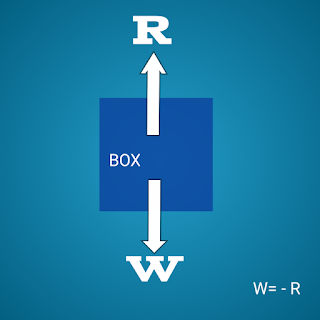### Mechanics // Laws of Motion // Newton's Second Law of Motion // Newton's Third Law of Motion // Action and Reaction // Impulse

1. Newton's Second Law of Motion

According to Newton's second law of motion the rate of change of momentum of a body with
respect to time is directly proportional to the net external force applied on it and the change
takes place in the direction of force. Second law defines force: a force is that which causes
an object to change the state of motion.
F = ma
where 'm' is the mass of the object and 'a' is the acceleration.

2. Newton's Third Law of Motion
The third law states that, to every action there is always an equal and opposite reaction.
According to this law, forces exist in a pair only because of action and reaction i.e.
isolated force does not exist in nature. But sometime, we may misinterpret the law and think
that if there are action and reaction acting on a body, the body must be at rest. But the action
and reaction forces do not act on the same body but act on two different bodies. Because of this
fact, action and reaction do not cancel to each other.
Let us exemplify the Newton's third law of motion.

(i) A block resting on a table: Because of the weight W of the block, it presses on the table by
its weight which is taken as action. The table also exerts force on the block equal in magnitude
but opposite in direction to that of the weight of the block called the reaction R. So the action
and reaction acts on two different bodies and hence W= - R.Figure : Weight of the block acting vertically downward on the table is equal to the normal reaction on the block acing upward.

(ii) Pushing a block: A man pushing a block on a smooth horizontal surface with negligible
friction as shown in Fig. 4.2(b). The man exerts a force Fi on the block and the block also
exerts a force F2 on the man. To move the block forward, the man must push backward against
the ground and the man gets the reaction force which is applied on the block. So the motion of
the block is possible. If there had been no friction, then it is not possible to move the block.

(iii) Swimming: While swimming in the pond, the swimmer pushes the water in backward
direction where as the reaction of the water pushes the swimmer in the forward direction. So
the swimmer can swim easily in the water.

(iv) Flight of a rocket: The burnt fuel comes out of the rocket in the form of hot and highly
compressed gases in the backward direction whereas the escaping gases push the rocket in
the forward direction.

(v) Walking: When we are walking on the road, we push the ground in the backward direction,
the man gets reaction force in forward direction.

3. Impulse
In some cases, a force acts for such a small time interval that we measure the effect of the force,
called the impulse. An impulsive force is not constant, but changes with time, first
from zero to maximum and then from maximum to zero. The variation of magnitude of the force
with respect to time is shown in the figure below. As the force is not constant, impulse is measured
by taking the product of the average force applied and the time interval for which the force is

Impulse = F(avg) * Time Interval

In other words, impulse of a force is measured by the change in linear momentum produced by
the force. It can be positive, negative or zero according as the momentum of the body increases,
decreases or remains same respectively.
Impulse is a vector quantity and takes the direction of force or the direction of change in momentum.
The SI-units of impulse is newton second or kg m/s.